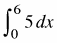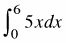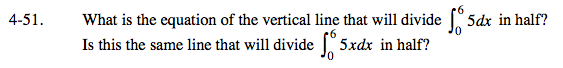### Home > CALC > Chapter 4 > Lesson 4.2.1 > Problem4-51

4-51.

What is the equation of the vertical line that will dividein half? Is this the same line that will dividein half? Homework Help ✎What is the area of that rectangle and where would you draw a vertical line to cut its area in half?

What is the area of that triangle and where would you draw a vertical line to cut its area in half?